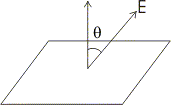# Electric field lines And Electric flux

## 8. Electric Field Lines

• For a single positive point charge q, electric field is
$$\boldsymbol{F}=\frac{kqq_{'}\boldsymbol{\widehat{r}}}{4\pi \epsilon _{0}r^{2}}$$
now to get feel of this field one can sketch a few representative vectors as shown in fig below• Since electric field varies as inverse of square of the distance that points from the charge the vector gets shorter as you go away from the origin and they always points radially outwards.

• Connecting up these vectors to form a line is a nice way to represent this field .

• The magnitude of the field is indicated by the density of the field lines.

• Magnitude is strong near the center where the field lines are close togather, and weak farther out, where they are relatively apart.

• So, electric field line is an imaginary line drawn in such a way that it's direction at any point is same as the direction of field at that point.

• An electric field line is, in general a curve drawn in such a way that the tangent to it ateach point is the direction of net field at that point.

• Field lines of a single position charge points radially outwards while that of a negative charge are radially inwards as shown below in the figure.• Field lines around the system of two positive charges gives a different picture and describe the mutual repulsion between them.• Field lines around a system of a positive and negative charge clearly shows the mutual attraction between them as shown below in the figure.• Some important general properties of field lines are
1.Field lines start from positive charge and end on a negative charge.
2.Field lines never cross each other if they do so then at the point of intersection there will be two direction of electric field.
3.Electric field lines do not pass through a conductor , this shows that electric field inside a conductor is always zero.
4.Electric field lines are continuous curves in a charge free region.

## 9. Electric Flux

• Consider a plane surface of area ΔS in a uniform electric field E in the space.

• Draw a positive normal to the surface and θ be the angle between electric field E and the normal to the plane.• Electric flux of the electric field through the choosen surface is then
Δφ = E ΔS cosθ

• Corresponding to area ΔS we can define an area vector ΔS of magnitude ΔS along the positive normal. With this definition one can write electric flux as
Δφ = E . ΔS

• direction of area vector is always along normal to the surface being choosen.

• Thus electric flux is a measure of lines of forces passing through the surface held in the electric field.

Special Cases
• If E is perpendicular to the surface i. e., parallel to the area vector then θ = 0 and
Δφ = E ΔS cos0

• If θ = π i. e., electric field vector is in the direction opposite to area vector then
Δφ = - E ΔS

• If electric field and area vector are perpendicular to each other then θ = π/2 and Δφ = 0

• Flux is an scaler quantity and it can be added using rules of scaler addition.

• For calculating total flux through any given surface , divide the surface into small area elements. Calculate the flux at each area element and add them up.

• Thus total flux φ through a surface S is
φ ≅ ΣE.ΔS

• This quantity is mathematically exact only when you take the limit ΔS→0 and the sum in equation 3 is written as integral
φ = ∫ΣE.dS

link to this page by copying the following text